×
Get Full Access to UMD - MATH 120 - Study Guide - Midterm
Get Full Access to UMD - MATH 120 - Study Guide - Midterm

×

UMD / Math / MATH 120 / What are the identities of factoring?

# What are the identities of factoring? Description

##### Description: This is a compilation of all the objectives that we have gone over in chapters 1 through 2 that are probably going to be on the midterm.
28 Pages 73 Views 1 Unlocks
Reviews

CALCULUS

CH. 1 + CH. 2

NOTES

(STUDY GUIDE)

CH. 0.3 THE ALGEBRA OF FUNCTIONS

Operation on Functions → Like f(x) + g(x), f(x) - g(x),, f(x)g(x)

• Basic properties of fractions
1. Multiplication a・=and=2. Simplifying common factors=3. Division==4. Addition=• Ex.         g(x) =h(x) =We also discuss several other topics like What does product configuration mean?

g(x) + h(x) =+, x ≠ 0, 1Don't forget about the age old question of What is the hill equation?

1. Find common denominator

() + ()

1. Multiply

=+1. Combine

=We also discuss several other topics like What do you think is the primary factor for soil production?

1. Simplify

=Composition of Functions (aka f(g(x)))

Way of combining 2 functions f(x) and g(x), substituting variable x with g(x) in f(x)

• Evaluating a Function
• Ex. If f(x) = x3, find, where h ≠ 0

1. Substitute

f(x+h) = (x+h)3 = x3 + 3x2h + 3xh2 + h3

1. Simplify

f(x+h) - f(x) = (x3 + 3x2h + 3xh2 + h3) - x3 = 3x2h + 3xh2 + h3If you want to learn more check out n2cl4

1. Factor H in Numerator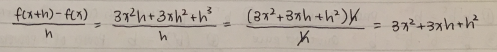We also discuss several other topics like presbyiatrics

CH. 0.4 ZEROS OF FUNCTIONS - QUADRATIC FORMULA + FACTORING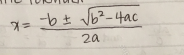• Finding zeros of Functions
• Ex. f(x) = 4x2 - 4x + 1

= 4x2 - 4x + 1 = 0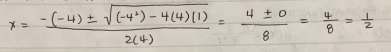• Finding Intersections of Graphs
• Ex. Finding the points of intersection of graphs : y = x2 + 1 and y = 4x

1. Equate two expressions for y (because coordinates must satisfy both eq.)

X2 + 1 = 4xDon't forget about the age old question of The communications technology is consist of?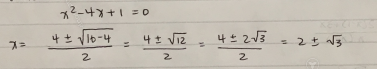1. Substitute x-values in equations to get y-values

Factoring

• Identities for factoring
1. Difference of squares                 A2 - B2 = (A-B) (A+B)
2. Perfect Square                A2 + 2AB + B2 = (A+B)2 or A2 - 2AB + B2 = (A-B)2
3. Difference of Cubes                A3 - B3 = (A-B) (A2 - AB + B2)
4. Sums of cubes                A3 + B3 = (A+B) (A2 - AB + B2)

CH. 0.5 Exponents + POwer Functions

Law of Exponents

1. brbs = br+s Product Rule
2. b-r =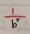Changing signs of exponents
3.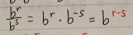Quotient Rule
4. (br)s = brs                 Power of a power
5. (ab)r = arbr                Power of a product
6.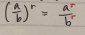Power of a Quotient

Simple Compound Interest :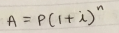Compound Interest w/ Multiple Interest Periods :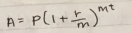WHERE P = principal amount (original amount deposited)

r = interest rate per annum

m = number of interest periods per year

t = number of years

i = compound interest rate per interest period

1.1 The Slope of a Straight Line

Slope-Intercept Equation        y = mx + b

Point-Slope Form                y - y, m(x-x,)

Parallel lines ➝ same slope

Perpendicular Lines ➝ opposite reciprocal

Be able to find:

• Slope of line through two points
• Equation of line given a point and a slope
• Equation of line through 2 points
• Equation of line parallel to a given line
• 1.3 The Derivation and Limits

Derivative of Linear Function : If mx + b = f(x), then f1(x) = m

Constant Rule                f(x) = b ➝ f1(x) = 0

Power Rule                f(x) = xr ➝ f1(x) = rxr-1

Page ExpiredIt looks like your free minutes have expired! Lucky for you we have all the content you need, just sign up here